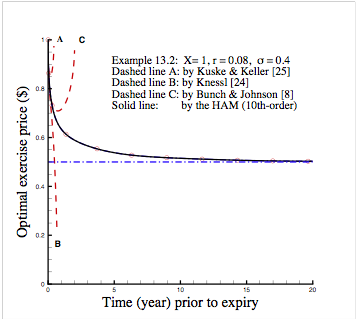Some Applications of the HAM

(1)  Nonlinear ODEs

Many nonlinear boundary-value/eigen-value problems governed by

F[u(x) , x] = 0

in a finite or an infinite interval can be solved by means of the Mathematica package BVPh 1.0.  Here, the governing equation may contain singularity and have multiple solutions, and the boundary condition may be satisfied at multiple points.

Many examples are given in Chapters 8 – 12 of Liao’s book: Homotopy Analysis Method in Nonlinear Differential Equations, Higher Education Press & Springer (2012)

(2)  American Put Option

American put option is governed by a partial differential equation (PDE) with an unknown moving boundary (i.e. the optimal exercise boundary).  The homotopy analysis method (HAM) is successfully applied to solve this famous problem in finance.  Unlike asymptotic and/or perturbation formulas that are often valid only a couple of days or weeks prior to expiry, the optimal exercise boundary given by the HAM may be valid a couple of dozen years, or even a half century!  This illustrates the great potential and general validity of the HAM for nonlinear PDE. A practical Mathematica package APOh with a simple user’s guide is provided for businessmen to gain accurate enough optimal exercise price of American put option at large expiration-time by a laptop only in a few seconds, which is free available at

For details, please refer to Chapter 13 of Liao’s book: Homotopy Analysis Method in Nonlinear Differential Equations, Higher Education Press & Springer (2012).(3)  Interaction of Nonlinear Water Wave and Non-uniform Current

In Chapter 15, we illustrate the validity of the homotopy analysis method (HAM) for a complicated nonlinear PDE describing the nonlinear interaction of a periodic traveling wave on a non-uniform current with exponential distribution of vorticity.  In the frame of the HAM, the original highly nonlinear PDE with variable coefficient is transferred into an infinite number of much simpler linear PDEs, which are rather easy to solve.  Physically, it is found that Stokes’ criterion of wave breaking is still correct for traveling waves on non-uniform currents.  It verifies that the HAM can be used to solve some complicated nonlinear PDEs so as to deepen and enrich our physical understanding about some interesting nonlinear phenomena.

For details, please refer to Chapter 15 of Liao’s book: Homotopy Analysis Method in Nonlinear Differential Equations, Higher Education Press & Springer (2012).

(4)  Resonance Criterion of Arbitrary Number of Nonlinear Water Waves

In Chapter 16, we verify the validity of the homotopy analysis method (HAM) for a rather complicated nonlinear PDE describing the nonlinear interaction of arbitrary number of traveling water waves.  In the frame of the HAM, the wave resonance criterion for arbitrary number of waves is gained, for the first time, which logically contains the famous Phillips’ criterion for four small amplitude waves.  Besides, it is found for the first time that, when the wave-resonance criterion is satisfied and the wave system is fully developed, there exist multiple steady-state resonant waves, whose amplitude might be much smaller than primary waves so that a resonant wave may contain much small percentage of the total wave energy.  This example illustrates that the HAM can be used as a tool to deepen and enrich our understandings about some rather complicated nonlinear phenomena.

For details, please refer to Chapter 16 of Liao’s book: Homotopy Analysis Method in Nonlinear Differential Equations, Higher Education Press & Springer (2012).